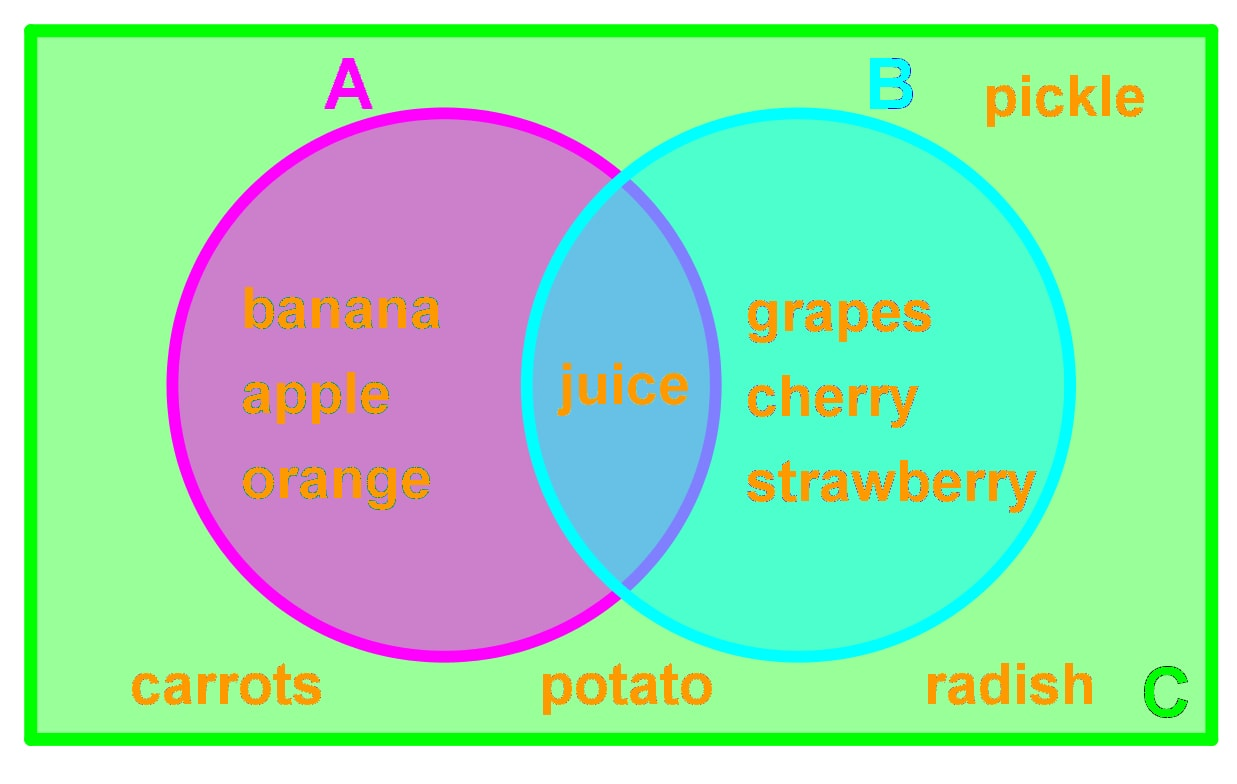# Intersection and union of 2 sets

### Intersection and union of 2 sets

#### Lessons

In this section we will learn about intersection and union of 2 sets.

Let A and B be sets. Then, the definitions for intersection and union is the following:

Intersection: A set of elements where the elements show up both in A and B. We call this intersection A$\cap$B. Sometimes people refer to the symbol $\cap$ as the word "and".

Union: A set of all elements that appears in A, in B, or both in A and B. We call this union A$\cup$B. Sometimes people refer to the symbol $\cup$ as the word "or".

Here is a definition that may be useful:

A\B: The set of elements that is in A but not in B. In short, it is just A minus B.

The principle of inclusion and exclusion of 2 sets says the following:

n(A$\cup$B) = n(A) + n(B) - n(A$\cap$B)

• Introduction
Introduction to Intersection and Union of 2 Sets
a)
Intersection of Two Sets

b)
Union of Two Sets

c)
A\B (A minus B)

d)
Principle of Inclusion and Exclusion

• 1.
Finding the Intersection & Union of 2 Sets

You are given the following Venn diagram:a)
Find A$\cap$C and n(A$\cap$C).

b)
Find A$\cup$C and n(A$\cup$C).

c)
Find C\A.

d)
Find (A$\cap$B)'

• 2.
Consider the following:

- Universal Set $U =$ {1, 2, 3, 4, 5, 6, 7, 8}

- Set A = {1,2,3,4,5,6}

- Set B = {2,4,6,8}

a)
Draw a Venn diagram to represent these sets.

b)
Find A$\cap$B and n(A$\cap$B).

c)
Find A$\cup$B and n(A$\cup$B).

d)
Find A\B.

e)
Find (A$\cup$B)'

• 3.
Consider the following:

- A = {$m | m = 4x, 0 \leq x \leq 3, x \in I$}

- B = {$n | n = 2x, 0 \leq x \leq 6, x \in I$}

a)
Draw a Venn diagram to represent these sets

b)
Find A$\cap$B and n(A$\cap$B).

c)
Find A$\cup$B and n(A$\cup$B).

d)
Find A\B.

• 4.
Using the Principle of Inclusion and Exclusion

Kevin asked 50 people if they liked to play soccer or basketball. 10 people didn't like either of them. 25 people liked soccer. 30 people liked basketball. How many people liked both soccer and basketball?

• 5.
Willy surveyed 30 people at a restaurant to see if they ordered noodles or rice. 10 people ordered both rice and noodles. 5 people only ordered a drink. 3 people ordered only rice.
a)
Draw a Venn diagram and label the missing information.

b)
Find the missing information using the principle of inclusion and exclusion.